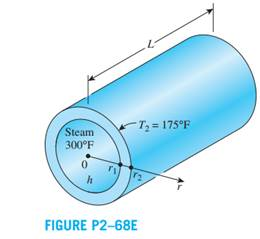Create an Account

Home / Questions / Consider a steam pipe of length L 30 ft inner radius r1 2 in outer radius r2 24 in and ...

Consider a steam pipe of length L 30 ft inner radius r1 2 in outer radius r2 24 in and thermal conductivity

Consider a steam pipe of length L = 30 ft, inner radius r1 = 2 in, outer radius r2 = 2.4 in, and thermal conductivity k = 7.2 Btu/h·ft·°F. Steam is flowing through the pipe at an average temperature of 300°F, and the average convection heat transfer coefficient on the inner surface is given to be h = 12.5 Btu/h·ft2·°F. If the average temperature on the outer surfaces of the pipe is T2 = 175°F,

(a) express the differential equation and the boundary conditions for steady one-dimensional heat conduction through the pipe,

(b) obtain a relation for the variation of temperature in the pipe by solving the differential equation, and (c) evaluate the rate of heat loss from the steam through the pipe.Jul 21 2020 View more View LessSubscribe To Get Solution